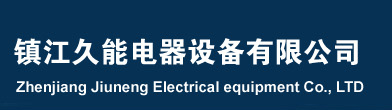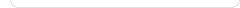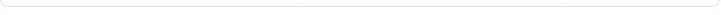## 自制点焊机雨什么缺点

40平方的铁芯在短时间内（暂载率35%）可达180A　日常使用足够了。

I II III. IN .NI 220V 380V

S=AxB（平方厘米）

E=4.44FBS*0.0001(V/匝）

F=50HZ（电源频率） B=1.1T-1.5T(硅钢片磁饱和感应强度)

__________

√2.78+0.167I2-1.67=(MM)

N2=N21+N22,N21:N22=1:3

S=AXB=8x6=48(cm2)

E=4.44fbsx0.0001=4.44x50x1.2x48x0.0001=1.2(V/匝)

N1=U1/E=220/1.2=183(匝)

N2=U2/E=60/1.2=50(匝)

N21=12(匝),N22=38(匝)

P1=3.5（E的2次方）=3.5x(1.2的2次方)=5(KVA)

P2=0.9P1=0.9x5=4.5(KVA)

I1=P1x1000/U1=5x1000/220=22.7(A)

I2=P2x1000/U2=4.4x1000/60=75(A)

____________

√2.78+0.167I2-1.67=(MM)

____________

√2.78+0.167x75-1.67=2.24(毫米)

1000元以上，那么可不可以自己做一台呢，

：价钱不过100元多点，不用电焊条。